# Addition & Subtraction of Mixed Numbers: With Same Denominators

##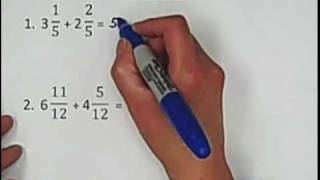By Red River College -Tutoring

Addition & Subtraction of Mixed Numbers: With Same Denominators# Adding and Subtracting Fractions with the Same Denominator

##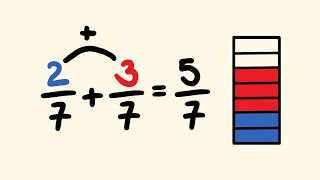By tecmath# [3.NF.3d-1.0] Compare Similar Fractions - Common Core Standard - Practice Problem

##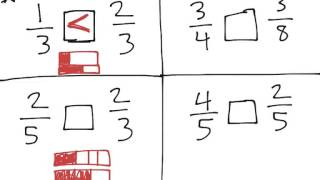By Freckle education

Compare two fractions with the same numerator or the same denominator by reasoning about their size# Comparing Fractions (3.NF.3D)

##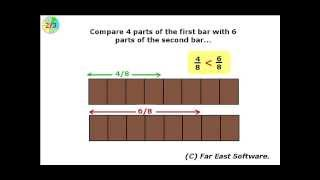By Far East Software

Compare two fractions with the same numerator or the same denominator by reasoning about their size# Common denominators

##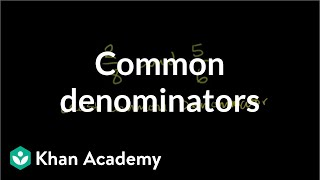By Khan Academy

Rewrite 2/8 and 5/6 to have the same denominator.# [4.NF.3b-1.0] Decomposing Fractions - Common Core Standard - Word Problem

##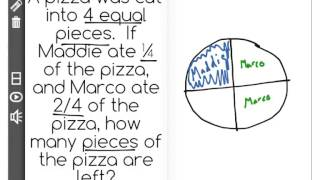By Freckle education

Decompose a fraction into a sum of fractions with the same denominator in more than one way# [4.NF.3b-1.0] Decomposing Fractions - Common Core Standard

##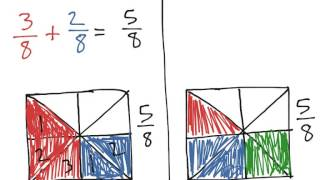By Freckle education

Decompose a fraction into a sum of fractions with the same denominator in more than one way# Renaming Fractions and Mixed Numbers

##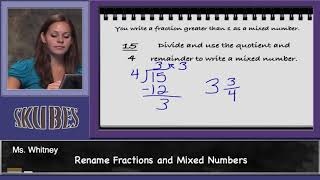By Skubes ed

Decompose a fraction into a sum of fractions with the same denominator in more than one way, recording each decomposition by an equation. Justify decompositions# Subtracting Fractions in a Word Problem

##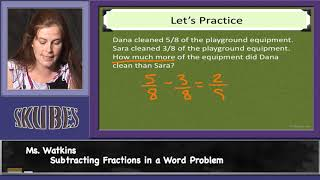By Skubes ed

Solve word problems involving addition and subtraction of fractions referring to the same whole and having like denominators# Multistep Fraction Problems

##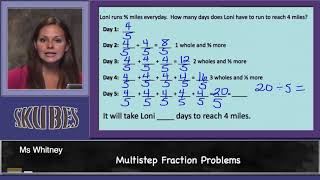By Skubes ed

Solve word problems involving addition and subtraction of fractions referring to the same whole and having like denominators# MULTIPLYING FRACTIONS WORD PROBLEMS

##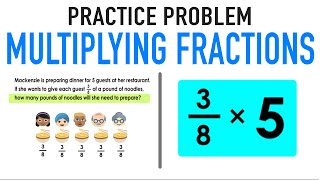By Mashup Math

Solve word problems involving addition and subtraction of fractions referring to the same whole and having like denominators# Comparing fractions with the same denominator | Math | 3rd grade | Khan Academy

##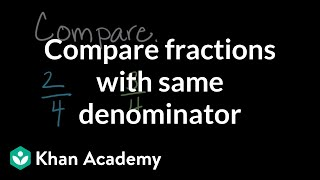By Khan Academy

compares one pair of fractions with visuals and another pair without visuals.# [4.NF.3d-1.0] Word Problems: Addition - Common Core Standard

##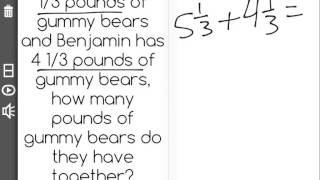By Freckle education

Solve word problems involving addition and subtraction of fractions referring to the same whole and having like denominators, e.g., by using visual fractions models and equations to represent to problem.# More on equivalent fractions | Fractions | 4th grade | Khan Academy

##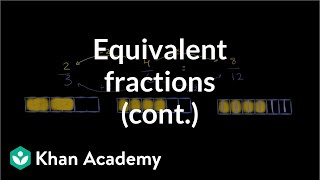By Khan Academy

Important: if you multiply a fraction's numerator and the denominator by the same number, you'll end up with an equivalent fraction. Got it? Maybe? No problem, we'll help!# Multiplying Integers | MathHelp.com

##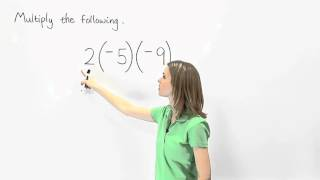By MathHelp.com

This lesson covers comparing fractions. Students learn to compare fractions with the same denominator, which are called like fractions, by comparing the numerators. For example, to compare 7/9 and 4/9, note that 7 is greater than 4, so 7/9 is greater than 4/9. Students also learn to compare fractions with the different denominators, which are called unlike fractions, by first finding a common denominator, then comparing the numerators. For example, to compare 1/2 and 1/3, first find a common denominator, or the Least Common Multiple of 2 and 3, which is 6. To get 6 in the denominator of 1/2, multiply the numerator and denominator by 3, to get 3/6. To get 6 in the denominator of 1/3, multiply the numerator and denominator by 2, to get 2/6. Next, compare 3/6 and 2/6. Note that 3 is greater than 2, so 3/6 is greater than 2/6, which means that 1/2 is greater than 1/3.# Comparing Fractions | MathHelp.com

##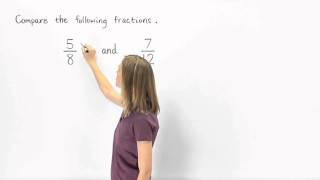By MathHelp.com

MathHelp.com offers comprehensive College Algebra help with a teacher. Are you stuck in a College Algebra class with a bad teacher and a huge classroom? Take the matter into your own hands with our custom College Algebra course. You will get through college math in no time!# Lowest Common Denominator | Subtracting Fractions | MathHelp.com

##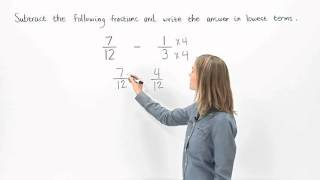By MathHelp.com##By MathHelp.com# [4.NF.2-1.0] Compare Dissimilar Fractions - Common Core Standard

##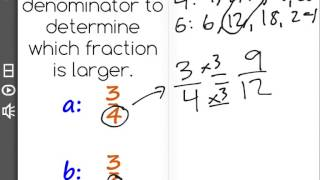By Freckle by Renaissance

Compare two fractions with different numerators and different denominators, e.g., by creating common denominators or numerators, or by comparing to a benchmark fraction such as 1/2. Recognize that comparisons are valid only when the two fractions refer to the same whole. Record the results of comparisons with symbols greater than , = , or less than , and justify the conclusions, e.g., by using a visual fraction model. Front Row is a free, adaptive, Common Core aligned math program for teachers and students in kindergarten through eighth grade. Front Row allows students to practice math at their own pace - learning advanced concepts when they're ready and receiving remediation when they struggle. Front Row provides teachers with access to a detailed data dashboard and weekly email reports that show which standards are causing students difficulty, what small groups can be formed for interventions, and how their students are progressing in math.# [4.NF.3b-1.0] Decomposing Fractions - Common Core Standard

##By Freckle by Renaissance

Decompose a fraction into a sum of fractions with the same denominator in more than one way, recording each decomposition by an equation and justify decompositions by using a visual fraction model. Front Row is a free, adaptive, Common Core aligned math program for teachers and students in kindergarten through eighth grade. Front Row allows students to practice math at their own pace - learning advanced concepts when they're ready and receiving remediation when they struggle. Front Row provides teachers with access to a detailed data dashboard and weekly email reports that show which standards are causing students difficulty, what small groups can be formed for interventions, and how their students are progressing in math.